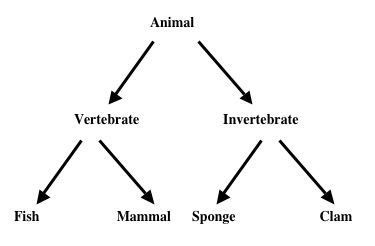# Type Labels II

Logical System

12/19/09

### Extending the notation

At first site, having an equivalence between types (lower case letters a..v) and monadic predicates (upper case A..V) might seem limiting. But it can be extending to a degree by introducing definitions for more complex single variable predicates. For example, say you have

(Mx∧Dx)⊃Nx  (*notice the free variable x*)

as a fancier category (those things which if they are M and D are also N), then you could introduce a monadic predicate definition for this, via an equivalence eg

(∀x)(Ax≡((Mx∧Dx)⊃Nx))

and then lower case 'a' would be your type. You'd have to include the definitions for your trees or derivations using this method.

Most logicians when they see a formula like

(Mx∧Dx)⊃Nx

wishing to be a predicate, will think it needs to be a lambda

(λx)((Mx∧Dx)⊃Nx)

The lambda notation is read 'I am a function of the variable x'. In this case (as we can tell from the component predicates) the function has truth values (ie true or false) as its values, so when this function gets applied to an object or term, say 'a', thus

(λx)((Mx∧Dx)⊃Nx)a

a truth value results (and which truth value depends on whether 'a' satisfies the conditions or not). So, in effect, this lambda expression is a predicate, it is an anonymous predicate; it does not have a name.

This approach could be followed. But it is slightly at odds with the intention to use types or type labels. Type labels are terms, they name things, whereas applied predicates make true or false statements; the first are terms, the second are well formed formulas.

There is a similar notation that would allow us to work with terms. In naive set theory, there are many equivalences between predicate like expressions and also many straight identity definitions between terms; what helps to bridge the two is the abstraction operator

{x: <scope>}

where the scope or body is an ordinary well formed formula, typically and normally with free occurrences of the variable x, for example

{x: ((Mx∧Dx)⊃Nx)}

This notation is read 'the x such that Mx and ...etc'. Abstractions are intended to be terms. So formulas like identities, between abstractions and other terms, are all well-formed formulas, eg

p={x: ((Mx∧Dx)⊃Nx)}

We can use abstractions as type labels, like this

(∀x:{x: ((Mx∧Dx)⊃Nx)})Mx

Such a type would be an anonymous type, it is a type label without a name. It is clearer to give it a name and introduce it by a definition (using an identity not an equivalence)

p={x: ((Mx∧Dx)⊃Nx)},
(∀x:p)Mx

Then Identity Elimination can be used in a derivation or a tree to substitute in for the type label on the quantified variable, to get for example

p={x: ((Mx∧Dx)⊃Nx)},
(∀x:p)Mx

(∀x:{x: ((Mx∧Dx)⊃Nx)})Mx

And a type label on a quantified variable will expand out exactly as you would expect it to

(∀x:{x: ((Mx∧Dx)⊃Nx)})Mx expands to the non-typed
(∀x)((Mx∧Dx)⊃Nx)⊃Mx)

### Type labels in use

Type labels are a notational simplification. They also support shortcuts to certain inference steps. What is needed first is a syntactic addition to allow statements that relate one type to another, in particular subtypes to supertypes. A common application of relating types is with any hierarchical classification system such as genus-species in biology, or a subject classification in knowledge representation. For example,We could certainly set about describing this by means of ordinary monadic predicates; for example,

let Ax be the symbolization of 'x is an animal'
let Vx be the symbolization of 'x is a vertebrate'
let Ix be the symbolization of 'x is an Invertebrate'

then

(∀x)(Vx⊃Ax)
(∀x)(Ix⊃Ax) etc.

But we could also have types (or type labels) for the predicates and write our statements

(∀x:v)Ax
(∀x:i)Ax etc.

But then we could go one step further and allow statements between types; for example,

(∀x)(Vx⊃Ax)

says that anything which is a vertebrate is an animal ie anything of type vertebrate is of type animal ie vertebrate is a subtype of animal; we could write this

v<a .

The statement 'v<a' is going to be a true or false statement in our now extended first order logic; it means

(∀x)(Vx⊃Ax)

and is true or false just as that statement is true or false.

And then we can improve some of our inference steps by allowing subtypes to 'inherit' from supertypes. For examples, let us take 'St. Francis is the patron saint of all animals' as a premise

(∀x)(Ax⊃Fx) let Fx mean 'St Francis is your patron saint'

We could write this

(∀x:a)Fx

and, given our classification hierarchy, we know (and could prove) that St Francis is the patron saint of Sponges etc. But we could short cut or quicken the inference steps in the proof if we allowed something like this.

(∀x:a)Fx ∧(s<a) ∴ (∀x:s)Fx

that is, there is a direct inference from supertypes to subtypes; the subtypes 'inherit' from supertypes. And this is the exact logical analog of inheritance in object oriented programming.

We will get to this with 'order sorted logic'.

To review. We can introduce type labels as abbreviations for (some) quantified monadic predicates. This will make some of the formalizations of English simpler and more natural. Then, if we allow statements relating types (ie subtype-supertype), we can make certain inferences, particularly those about classification systems, quicker and more efficient. There is a point to note. Our logic with type labels still has just one domain or universe that the variables range over, exactly like ordinary first order logic; it is just that the type labels allow us to pick out certain elements of the universe (those satisfying the types).; many-sorted logic, to be described shortly, is different to this.